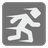Achilles and the Tortoise
Elementary
English ES JA RU

In a race, the quickest runner can never overtake the slowest, since the pursuer must first reach the point whence the pursued started, so that the slower must always hold a lead.

– as recounted...

A1 is faster than T2, so it has a X seconds head start on A1. For X seconds T2 will move at t2_speed*X metres. So A1 must first reach the point whence T2 already reached. But T2 is moving and next step for A1 is to reach the next point and so on to infinity. The paradox is correct in theory, but in practice A1 easily outruns T2. Hm... maybe we can calculate when A1 will catch up to T2.You are given A1 and T2’s speed in m/s as well as the length of the advantage T2 has in seconds. Try to count the time when from when A1 come abreast with T2 (count from T2 start). The result should be given in seconds with precious ±10-8.

Input: Three arguments. Speeds of A1 and T2 and advantage as integers.

Output: The time when A1 catch up T2 (count from T2 start) as an integer or float.

Example:

```chase(6, 3, 2) == 4
chase(10, 1, 10) == 11.11111111
```

How it is used: Let's return to school and remember our basic math principles. Sometimes simple arithmetic allows us to resolve difficult paradox problems easily.

Precondition:
t2_speed < a1_speed < 343PyCharm The Python IDE for Professional Developers. Enjoy productive Python, web and scientific development with PyCharm. Download it now!